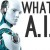## 深度学习

#### sklearn中的Bunch数据类型

<class 'sklearn.utils.Bunch'>

<class 'sklearn.utils.Bunch'>

#### sklearn中SGDClassifier分类器每次得到的结果都不一样？

sgdc.fit(X_train,y_train)
sgdc_predict_y = sgdc.predict(X_test)
print 'Accuary of SGD classifier ', sgdc.score(X_test,y_test)
print classification_report(y_test,sgdc_predict_y,target_names=['Benign','Malignant'])

SGDClassifier(random_state = None)

```sgdc = SGDClassifier()
sgdc.fit(X_train,y_train)
sgdc_predict_y = sgdc.predict(X_test)
print 'Accuary of SGD classifier ', sgdc.score(X_test,y_test)
print classification_report(y_test,sgdc_predict_y,target_names=['Benign','Malignant'])```

SGDClassifier(random_state = None)

#### sklearn中的Bunch数据类型

<class 'sklearn.utils.Bunch'>

<class 'sklearn.utils.Bunch'>

#### sklearn中SGDClassifier分类器每次得到的结果都不一样？

sgdc.fit(X_train,y_train)
sgdc_predict_y = sgdc.predict(X_test)
print 'Accuary of SGD classifier ', sgdc.score(X_test,y_test)
print classification_report(y_test,sgdc_predict_y,target_names=['Benign','Malignant'])

SGDClassifier(random_state = None)

```sgdc = SGDClassifier()
sgdc.fit(X_train,y_train)
sgdc_predict_y = sgdc.predict(X_test)
print 'Accuary of SGD classifier ', sgdc.score(X_test,y_test)
print classification_report(y_test,sgdc_predict_y,target_names=['Benign','Malignant'])```

SGDClassifier(random_state = None)